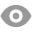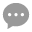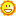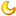# 射击之星K线形态测试和代码版本汇总99 |4 |王博 | 2020-4-7 22:49:22 | 显示全部楼层 |阅读模式
 1)射击之星什么意思 形态条件1：形态在上涨过程中出现量化定义：当日收盘价>20日均线形态条件2：形态上影线较长，下影线较短量化定义：上影线>5倍的下影线 形态条件3：形态上影线比实体长量化定义：上影线>实体 2) 测试买卖条件买入条件：出现射击之星收盘前，买入卖出条件：买入后的第二天收盘前，卖出

 测试一代码： //定义仓位 FUND:=10000000; LOTS:=INTPART(FUND*0.8/(CLOSE+FEE));//计算手数，使每次开仓金额都是800W //定义20日均线 MA20:=MA(C,20); //定义K线 KXST:=ABS(O-C);//定义实体 SYXCD:=H-MAX(O,C);//定义上影线 XYXCD:=MIN(O,C)-L;//定义下影线 //定义射击之星 SJZX1:=SYXCD>XYXCD*5;//上影线是下影线的5倍 SJZX2:=SYXCD>KXST;//上影线长度大于实体长度 SJZX3:=C>MA(C,20);//定义上涨趋势 SJZX:=SJZX1 AND SJZX2 AND SJZX3; SJZX, BK(LOTS);//射击之星当天买入 BARSBK=1,SP(BKVOL);//1天后卖出

 测试二代码： //定义仓位 FUND:=10000000; LOTS:=INTPART(FUND*0.8/(CLOSE+FEE));//计算手数，使每次开仓金额都是800W //定义60日均线 MA60:=MA(C,60); //定义K线 KXST:=ABS(O-C);//定义实体 SYXCD:=H-MAX(O,C);//定义上影线 XYXCD:=MIN(O,C)-L;//定义下影线 //定义射击之星 SJZX1:=SYXCD>XYXCD*5;//上影线是下影线的5倍 SJZX2:=SYXCD>KXST;//上影线长度大于实体长度 SJZX3:=C>MA(C,60);//定义上涨趋势 SJZX:=SJZX1 AND SJZX2 AND SJZX3; SJZX, BK(LOTS);//射击之星当天买入 BARSBK=1,SP(BKVOL);//买入1天后卖出

 测试三代码： //定义仓位 FUND:=10000000; LOTS:=INTPART(FUND*0.8/(CLOSE+FEE));//计算手数，使每次开仓金额都是800W //定义20日均线 MA20:=MA(C,20); //定义K线 KXST:=ABS(O-C);//定义实体 SYXCD:=H-MAX(O,C);//定义上影线 XYXCD:=MIN(O,C)-L;//定义下影线 //定义射击之星 SJZX1:=SYXCD>XYXCD*5;//上影线是下影线的5倍 SJZX2:=SYXCD>KXST;//上影线长度大于实体长度 SJZX3:=C>MA(C,20);//定义上涨趋势 SJZX:=SJZX1 AND SJZX2 AND SJZX3; SJZX, BK(LOTS);//射击之星当天买入 BARSBK=3,SP(BKVOL);//1天后卖出

 测试四代码： //定义仓位 FUND:=10000000; LOTS:=INTPART(FUND*0.8/(CLOSE+FEE));//计算手数，使每次开仓金额都是800W //定义20日均线 MA20:=MA(C,20); //定义DMI指标 DDD:=MA(C,10)-MA(C,50);//短周期收盘价均值与长周期收盘价均值做差 AMA:=MA(DDD,10);//M个周期的DDD均值 DDD1:=DDD>AMA; //定义K线 KXST:=ABS(O-C);//定义实体 SYXCD:=H-MAX(O,C);//定义上影线 XYXCD:=MIN(O,C)-L;//定义下影线 SJZX1:=SYXCD>XYXCD*5;//上影线是下影线的5倍 SJZX2:=SYXCD>KXST;//上影线长度大于实体长度 SJZX3:=C>MA(C,20);//定义上涨趋势 SJZX:=SJZX1 AND SJZX2 AND SJZX3; SJZX AND DDD1, BK(LOTS);//出现射击之星，并且DDD>AMA时，买入 BARSBK=1,SP(BKVOL);//1天后卖出

 您需要登录后才可以回帖 登录 | 立即注册 本版积分规则 回帖后跳转到最后一页79主题 1081积分1081